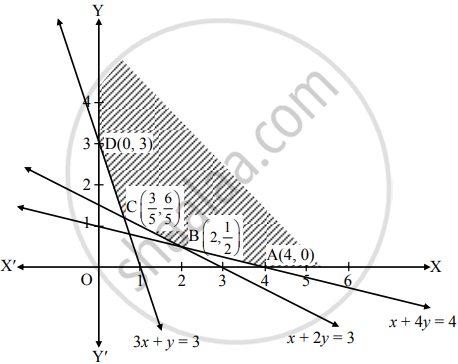Advertisement Remove all ads

# Solve the following L.P.P. by graphical method : Minimize: Z = 6x + 2y subject to x + 2y ≥ 3, x + 4y ≥ 4, 3x + y ≥ 3, x ≥ 0, y ≥ 0. - Mathematics and Statistics

Graph

Solve the following L.P.P. by graphical method :

Minimize: Z = 6x + 2y subject to x + 2y ≥ 3, x + 4y ≥ 4, 3x + y ≥ 3, x ≥ 0, y ≥ 0.

Advertisement Remove all ads

#### Solution

To draw the feasible region, construct table as follows:

 Inequality x + 2y ≥ 3 x + 4y ≥ 4 3x + y ≥ 3 Corresponding equation (of line) x + 2y = 3 x + 4y = 4 3x + y = 3 Intersection of line with X-axis (3, 0) (4, 0) (1, 0) Intersection of line with Y-axis (0, 3/2) (0, 1) (0, 3) Region Non-origin side Non-origin side Non-origin side

Shaded portion XABCDY is the feasible region,
whose vertices are A(4, 0), B, C and D(0, 3).
B is the point of intersection of the lines x + 4y = 4 and x + 2y = 3
Solving the above equations, we get

x = 2, y = (1)/(2)    ∴ B ≡ (2, 1/2)

C is the point of intersection of the lines x + 2y = 3 and 3x + y = 3.
Solving the above equations, we get

x = (3)/(5), y = (6)/(5)  ∴ C ≡ (3/5, 6/5)Here, the objective function is Z = 6x + 2y
Z at A(4, 0) = 6(4) + 2(0) = 24

Z at B(2, 1/2) = 6(2) + 2(1/2) = 12 + 1 = 13

Z at C(3/5, 6/5) = 6(3/5) + 2(6/5) = (18)/(5)) + (12)/(5) = 6

∴ Z at D(0, 3) = 6(0) + 2(3) = 6

∴ Z has minimum value 6 at C(3/5, 6/5) and D(0, 3).

∴ Z is minimum when, x = (3/5), y = (6/5), Z = 6 and x = 0, y = 3, Z = 6.

Is there an error in this question or solution?
Advertisement Remove all ads

#### APPEARS IN

Advertisement Remove all ads
Advertisement Remove all ads
Share
Notifications

View all notifications

Forgot password?